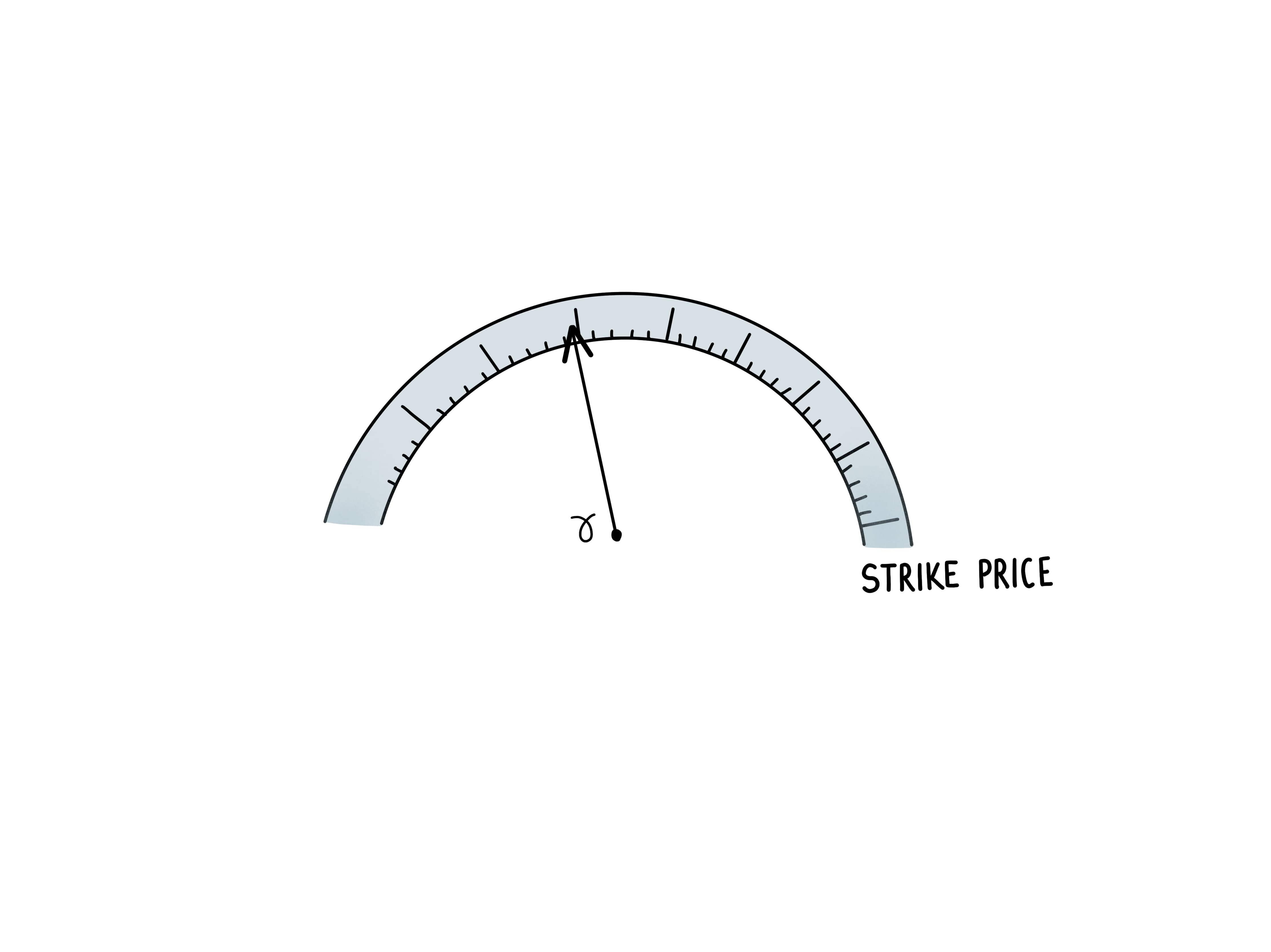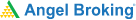# Option Greek: Know All about Gamma## Say Hello to Gamma

In the previous chapters, we were introduced to the concepts of Option Greeks, under which we learned about Delta. If you remember, Delta measures the sensitivity of the option price to changes in the price of the underlying asset. There is another Option Greek called Gamma.

Option Gamma measures the sensitivity of changes in delta to a unit change in the underlying stock price. Delta is a dynamic number that changes in response to aspects such as volatility, time to expiry and interest rates. To understand the degree to which Delta changes in response to these various stimuli, we use Gamma. In the following sections, we will explore Gamma, Gamma trading and how to use gamma in option trading.

### Understanding Gamma Movements

Gamma is expressed as a change in the delta per one point change in the price of the underlying. For instance, if the futures price is 200, a 220 call has a delta of 30 and a gamma of 2. If the futures price increases to 201, the delta is now 32. Conversely, if the futures price decreased to 199, the delta is 28. Just like delta, gamma too is dynamic. Gamma is the highest when the underlying price is near the option’s strike price.

The gamma decreases as the underlying moves away from the strike price and increases as it moves towards strike price. Gamma is highest in at-the-money options as their deltas are most sensitive to underlying price changes.

### Calculating Gamma

Gamma is calculated as the difference in delta divided by the change in underlying price. For instance, you have an underlying futures contract at 200 and the strike price is 200. The options delta is 50 and the options gamma is 3. If the futures price moves to 201, the options delta is changed to 53. If the futures price moves down to 199, the options delta is 47.

Across the two-point underlying futures contract move, the delta changed by 6.

### Understanding all About Gamma of Options

Delta is a measure of the rate of change in the option premium whereas gamma measures the momentum. This is how gamma measures the movement risk. Like delta, the gamma too ranges between 0 and 1. Gammas are linked to whether your option is long or short in the market. So if you are long on a call option or long on a put option then your gamma will be positive. Whereas, if you are short on a call option or short on a put option then your gamma will be negative.

Learning & Earning is now super simple₹ 0 Equity Delivery

No Hidden Charges₹ 20 Per Order For Intraday

FAQ,Currencies & CommoditiesZERO Brokerage*

on ALL SegmentsFREE Margin

Trade Funding

### Understanding gamma with an example

Like we learned before, gamma is normally the maximum when the strike price is very close to the stock price i.e. in case of ATM options. That is also the time when the impact on the delta is the maximum. We also saw that delta shifts the maximum when the option is ATM whereas it shifts slightly at ITM and OTM. As the options become very deep ITM or deep OTM, the impact on delta is minimal. This is precisely what the gamma curve will reflect. It will look like a bell shaped curve where the gamma will be the maximum around the ATM options, whereas it will become flatter as you go deep OTM or deep ITM, the bell curve starts becoming flat.

Let’s take an example to understand this better.

Let’s assume that a stock is quoting at Rs. 850 and there is an OTM 870 call option that is quoting at Rs.18. Let us also assume that this stock has a delta of 0.4(40%) and a gamma of 0.1(10%). What happens when the stock price moves up from Rs.850 to Rs.880? Let us look at the impact on the option price (using delta) and on the delta (using gamma).

Since the delta is 0.4, the call option price will move up by 0.4 x (30) {Delta times change in the price of the underlying}. Thus the 870 call option price will move up by Rs.12 from Rs.18 to Rs.30.

In this case, what happens to the delta? The delta moves up by the extent of the gamma! This is because the gamma measures the sensitivity of the delta to shifts in the stock price.

#### Wrapping Up

Finally, let’s find out what impacts the value of the gamma.

The value of gamma is extremely dynamic and keeps changing over time. There are two factors that impact the value of the gamma over a period of time:

1. Time to expiry
2. Volatility of the stock price

Let’s explore how these two factors affect Gamma.

The gamma of the ATM option witnesses an increase as the time to expiration draws closer. At the same time, the gamma OTM and ITM options reduce. This occurs because there is greater scope for shift in delta in ATM options in comparison to deep OTM and ITM. The impact of expiration time on gamma values can be understood in another way.

Let’s take three options with terms to maturity of 1 month, 2 months and 3 months respectively. While all the 3 options will have a bell-shaped gamma curve, the bell curve will be sharpest in case of the 1 month option and the flattest for the 3 month option. That is because there is a greater visibility of change in delta in the 1 month option as compared to the 3 month option, which is still a bit hazy.

Let’s look at two situations where volatility is high and another situation where the volatility is low. Can you guess what the gamma curve will look like? That’s right!  The gamma curve will be flat in case of high volatility and it will be sharp in case of low volatility. This happens because when the volatility is high, there is a good degree of time value that is priced into the options. Hence, the scope for changes in delta is limited, making the gamma curve much flatter in times of high volatility.

#### A Quick Recap

1. Option Gamma measures the sensitivity of changes in delta to a unit change in the underlying stock price.
2. The gamma decreases as the underlying moves away from the strike price and increases as it moves towards strike price.
3. Gamma is highest in at-the-money options as their deltas are most sensitive to underlying price changes.
4. Gamma is calculated as the difference in delta divided by the change in underlying price.
5. Like delta, the gamma too ranges between 0 and 1.
6. So if you are long on a call option or long on a put option then your gamma will be positive.
7. If you are short on a call option or short on a put option then your gamma will be negative.
8. There are two factors that impact the value of the gamma over a period of time: time to expiry and volatility of the stock price.Test Your Knowledge

Take the quiz for this chapter & mark it complete.

Comments (0)

Add Comment

Get Information Mindfulness!

Catch-up With Market

News in 60 Seconds.The perfect starter to begin and stay tuned with your learning journey anytime and anywhere.Ready To Trade? Start withOpen an account